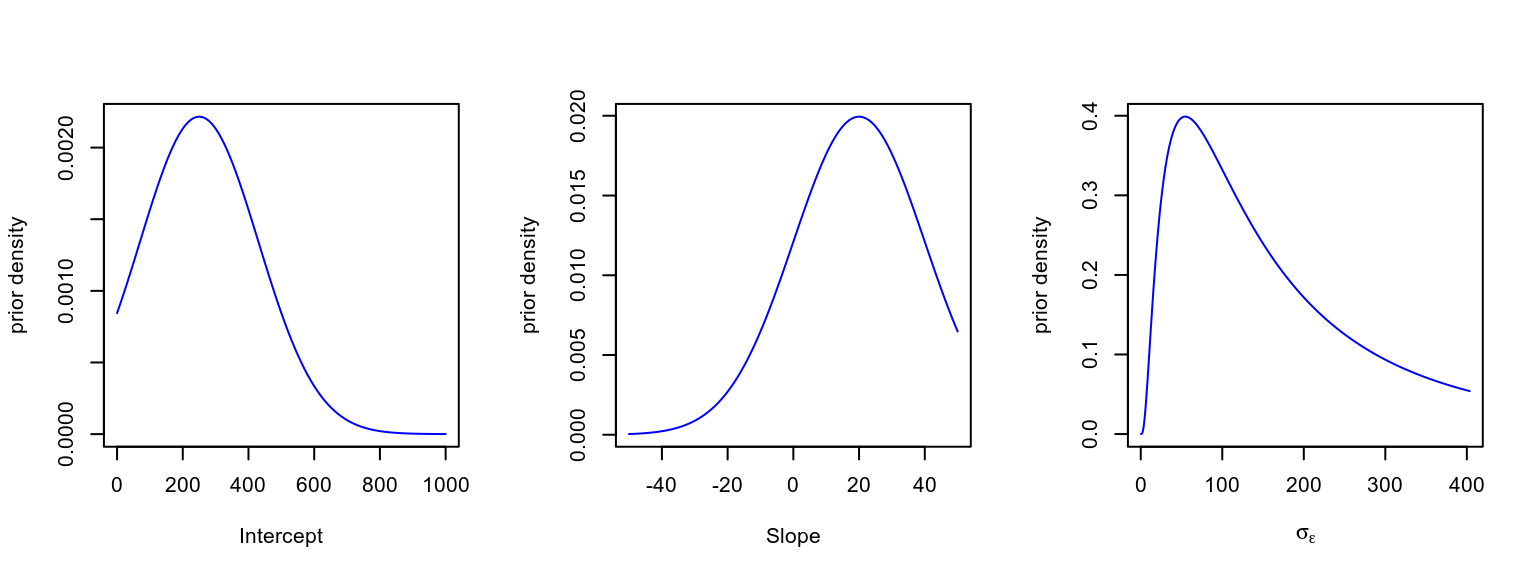GDR Vision - Paris 4/10/2018

## Today's program

1. Multilevel models from a frequentist perspective
2. The Bayesian approach
3. Markov Chain Monte Carlo (MCMC)
4. Examples

## Linear models

• The dependent variable $$y$$ is modeled as a weighted combination of the independent variables, plus an additive error $$\epsilon$$ $y_i=\beta_0 + \beta_1x_{1i} + \ldots +\beta_nx_{ni} + \epsilon_i \\ \epsilon \sim \mathcal{N}\left( 0, \sigma^2 \right)$

## Linear models

• The dependent variable $$y$$ is modeled as a weighted combination of the independent variables, plus an additive error $$\epsilon$$ $\textbf{Y} = \textbf{X}\beta + \epsilon \\ \epsilon \sim \mathcal{N}\left( 0, \sigma^2 \right)$

Matrix notation:

$\bf{Y} = \bf{X}\beta + \epsilon$ $\left( \begin{array}{c} y_1 \\ \vdots \\ y_m \end{array} \right) = \left( \begin{array}{cccc} 1 & x_{11} & \ldots & x_{1n}\\ \vdots & \vdots & \vdots & \vdots\\ 1 & x_{m1} & \ldots & x_{mn} \end{array} \right) \left( \begin{array}{c} \beta_0 \\ \beta_1 \\ \vdots \\ \beta_n \end{array} \right) + \left( \begin{array}{c} \epsilon_1 \\ \vdots \\ \epsilon_m \end{array} \right)$

Matrix multiplication: $\left( \begin{array}{cc} a & b \\ c& d \end{array} \right) \left( \begin{array}{c} 1 \\ 2 \end{array} \right) = 1 \left( \begin{array}{c} a \\ c \end{array} \right) + 2 \left( \begin{array}{c} b \\ d \end{array} \right) = \left( \begin{array}{c} a + 2b \\ c+ 2d \end{array} \right)$

## Linear mixed-effects models

• 'Classical' linear models are fixed-effects only:
• independent variables are all experimental manipulation (they are not random).
• the only random source of variation is the residual error $$\epsilon \sim \mathcal{N}\left( 0, \sigma^2 \right)$$.
• However observations (e.g. trials) are often grouped according to observational cluster (e.g. subjects), random samples from a larger population, on which we'd like to make inferences.
• In order to properly generalize from the sample to the population, these variables should be treated as random effects. Mixed-effects models allow to do that by explicitly modelling the population distribution.
• A model containing both fixed and random effects is usually called a mixed-effects model (but also: hierarchical, multilevel, etc.).
• Random effects are treated as random variations around a population mean. These random variations are usually assumed to have a Gaussian distribution.
• A simple example: a random-intercept model. Regressions have the same slope in each of the $$J$$ groups ($$j=1,\dots,J$$), but random variations in intercept $y_{ij} = \beta_0 + b_j + \beta_1x_{ij} + \epsilon_{i}\\ \epsilon \sim \mathcal{N}\left( 0, \sigma^2 \right)\\ b \sim \mathcal{N}\left( 0, \sigma_b^2 \right)$
• General formulation in matrix notation $\textbf{Y} = \textbf{X}\beta + \textbf{Z}b + \epsilon$ where $$\textbf{X}$$ and $$\textbf{Z}$$ are the known fixed-effects and random-effects regressor matrices.

• The components of the residual error vector $$\epsilon \sim \mathcal{N}\left( 0, \sigma^2 \right)$$ are assumed to be i.i.d. (independent and identically distributed).

• The random-effect components, $$b \sim \mathcal{N}\left( 0, \Omega \right)$$ are assumed to be normally distributed with mean 0, however they are not necessarily independent (the components $$b_j$$ can be correlated, and correlations can be estimated). Example:$\left[ {\begin{array}{c} {{b_0}}\\ {{b_1}} \end{array}} \right] \sim\cal N \left( {\left[ {\begin{array}{c} 0 \\ 0 \end{array}} \right],\Omega = \left[ {\begin{array}{c} {{\mathop{\rm Var}} \left( b_0 \right)} & {{\mathop{\rm cov}} \left( {{b_0},{b_1}} \right)}\\ {{\mathop{\rm cov}} \left( {{b_0},{b_1}} \right)} & {{\mathop{\rm Var}} \left( b_1 \right)} \end{array}} \right]} \right)$

## Likelihood function

• Parameters ($$\beta$$, $$\sigma^2$$ and $$\Omega$$) are estimated by maximizing the likelihood function, which is the probability of the data, given the parameters (but treated as a function of the parameters, keeping the data fixed).

• The probability of the data conditional to the random effects is integrated with respect to the marginal density of the random effects, to obtain the marginal density of the data $L\left(\beta,\sigma^2,\Omega \mid \text{data}\right) = \int p \left(\text{data} \mid \beta,\sigma^2, b\right) \, p\left(b \mid \Omega\right) \, db$

## ML vs REML

• The $$\textsf{R}$$ library lme4 provides two methods for estimating parameters: Maximum Likelihood (ML) and Restricted Maximum Likelihood (REML).

• ML tend to be biased and underestimate the variance components (e.g. $$\Omega$$).

• REML provide less biased variance estimates: conceptually can be seen as similar to Bessel's correction for sample variance (using $$n-1$$ instead of $$n$$ in the denominator).

• Improved estimates for repeated sampling (e.g. repeated-measures design).
• Improved estimates for imbalances in sampling (e.g. unequal number of trials across subjects).
• Avoid averaging (pre-averaging of data remove variation and can manifacture false confidence).
• Subject-specific standard error is taken into account in group-level estimates.
• Variation among group or individuals is modelled explicitly.
• Outperform classical methods in predictive ability.

## Example 1 sleepstudy

sleepstudy is a dataset in the lme4 package, with reaction times data from 18 subjects that were restricted to 3 hours of sleep for 10 days.

Questions:

• how reaction times changes with each sleep-deprived night?
• are individual difference in baseline response times related to individual differences in the effect of sleep deprivation?
str(sleepstudy)
'data.frame':   180 obs. of  3 variables:
$Reaction: num 250 259 251 321 357 ...$ Days    : num  0 1 2 3 4 5 6 7 8 9 ...
$Subject : Factor w/ 18 levels "308","309","310",..: 1 1 1 1 1 1 1 1 1 1 ...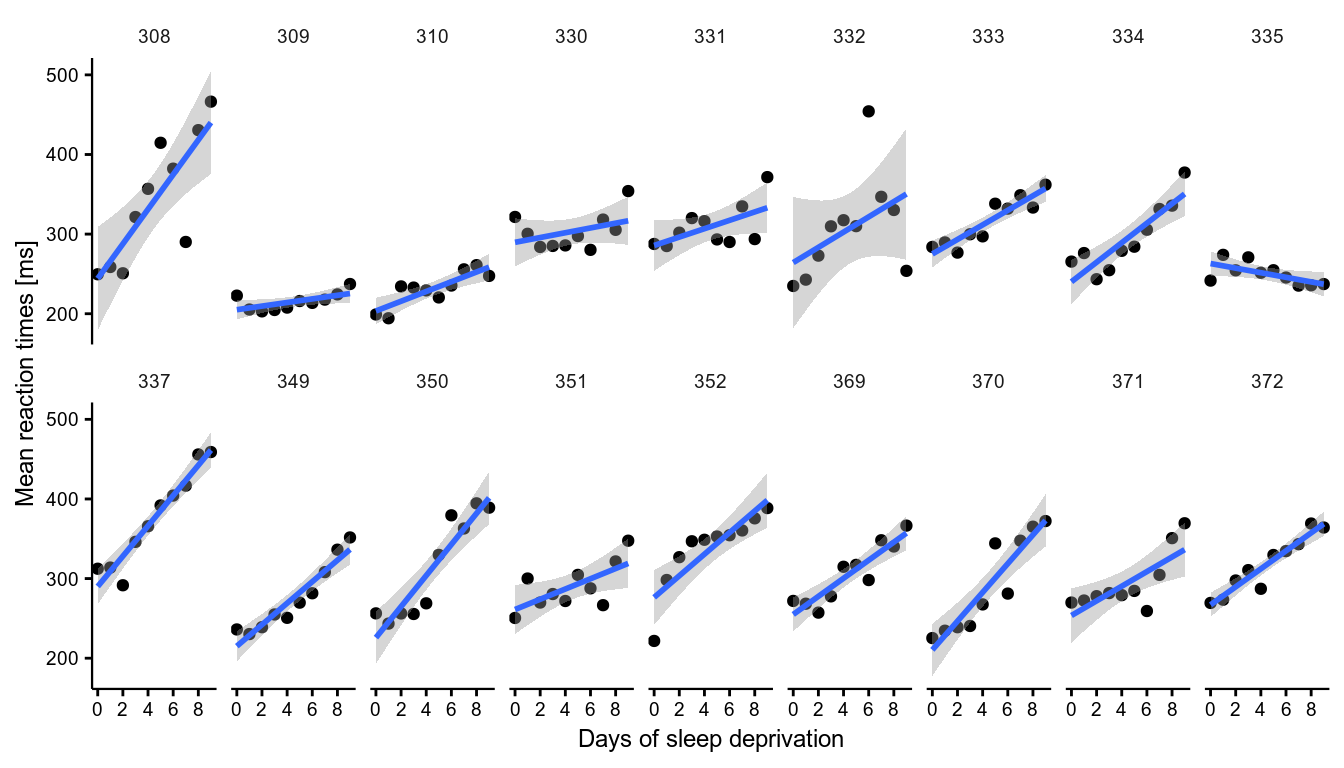• The model we want to fit includes both random slopes and intercept $y_{ij} = \beta_0 + b_{0j} + \left( \beta_1 + b_{1j} \right) \times {\rm{Days}}_i + \epsilon_i \\ \epsilon \sim \mathcal{N}\left( 0, \sigma^2 \right) \\ b\sim\cal N \left( 0, \Omega\right)$ • In lme4 m.1 <- lmer(Reaction ~ 1 + Days + (1 + Days | Subject), sleepstudy) summary(m.1) Linear mixed model fit by REML ['lmerMod'] Formula: Reaction ~ 1 + Days + (1 + Days | Subject) Data: sleepstudy REML criterion at convergence: 1743.6 Scaled residuals: Min 1Q Median 3Q Max -3.9536 -0.4634 0.0231 0.4634 5.1793 Random effects: Groups Name Variance Std.Dev. Corr Subject (Intercept) 612.09 24.740 Days 35.07 5.922 0.07 Residual 654.94 25.592 Number of obs: 180, groups: Subject, 18 Fixed effects: Estimate Std. Error t value (Intercept) 251.405 6.825 36.84 Days 10.467 1.546 6.77 Correlation of Fixed Effects: (Intr) Days -0.138 The function confint() allow easily to compute bootstrapped 95% CI of the parameters CI_fixef <- confint(m.1, method = "boot", nsim = 500, oldNames = F) print(CI_fixef, digits = 2) 2.5 % 97.5 % sd_(Intercept)|Subject 12.81 35.63 cor_Days.(Intercept)|Subject -0.53 0.81 sd_Days|Subject 3.57 8.32 sigma 22.61 28.44 (Intercept) 237.02 264.76 Days 7.54 13.20 • Tests of fixed and random effects can also be conducted using likelihood ratio tests, by comparing the likelihood of two nested models. • If $$L_1$$ and $$L_2$$ are the maximised likelihoods of two nested models with $$k_1 < k_2$$ parameters, the test statistic is $$2\text{log}\left( L_2/ L_1 \right)$$, which is approximately $$\chi^2$$ with $$k_2 - k_1$$ degrees of freedom. • Example: test if there are substantial variation across subjects in the effect of Days # m.1 <- lmer(Reaction ~ 1 + Days + (1 + Days|Subject), # sleepstudy) m.2 <- lmer(Reaction ~ 1 + Days + (1 | Subject), sleepstudy) anova(m.1, m.2) refitting model(s) with ML (instead of REML) Data: sleepstudy Models: m.2: Reaction ~ 1 + Days + (1 | Subject) m.1: Reaction ~ 1 + Days + (1 + Days | Subject) Df AIC BIC logLik deviance Chisq Chi Df Pr(>Chisq) m.2 4 1802.1 1814.8 -897.04 1794.1 m.1 6 1763.9 1783.1 -875.97 1751.9 42.139 2 7.072e-10 *** --- Signif. codes: 0 '***' 0.001 '**' 0.01 '*' 0.05 '.' 0.1 ' ' 1 Shrinkage: the predicted $${\hat b}_j$$ (conditional modes of the random effects) can be seen as a "compromise" between the within-subject estimates and the population mean.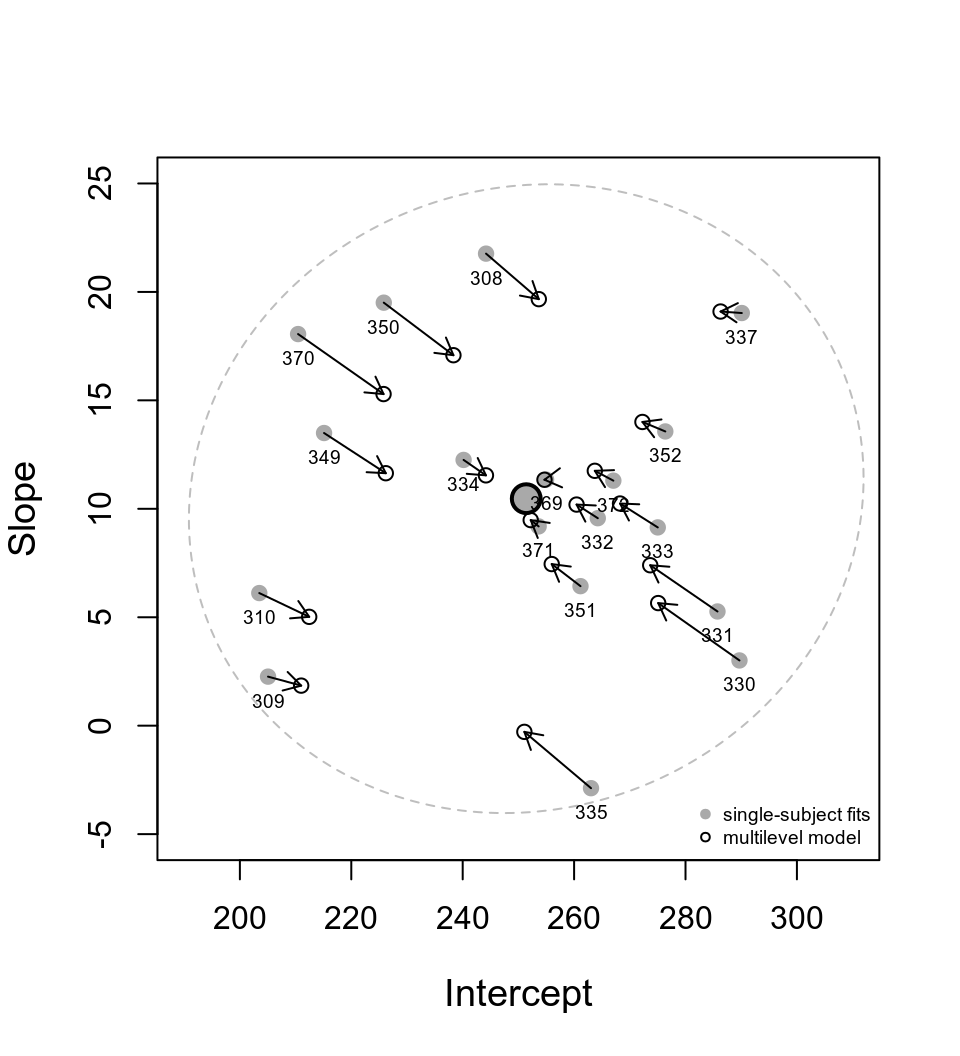## Diagnostic As for any linear model, it is important to check that residual errors are well-behaved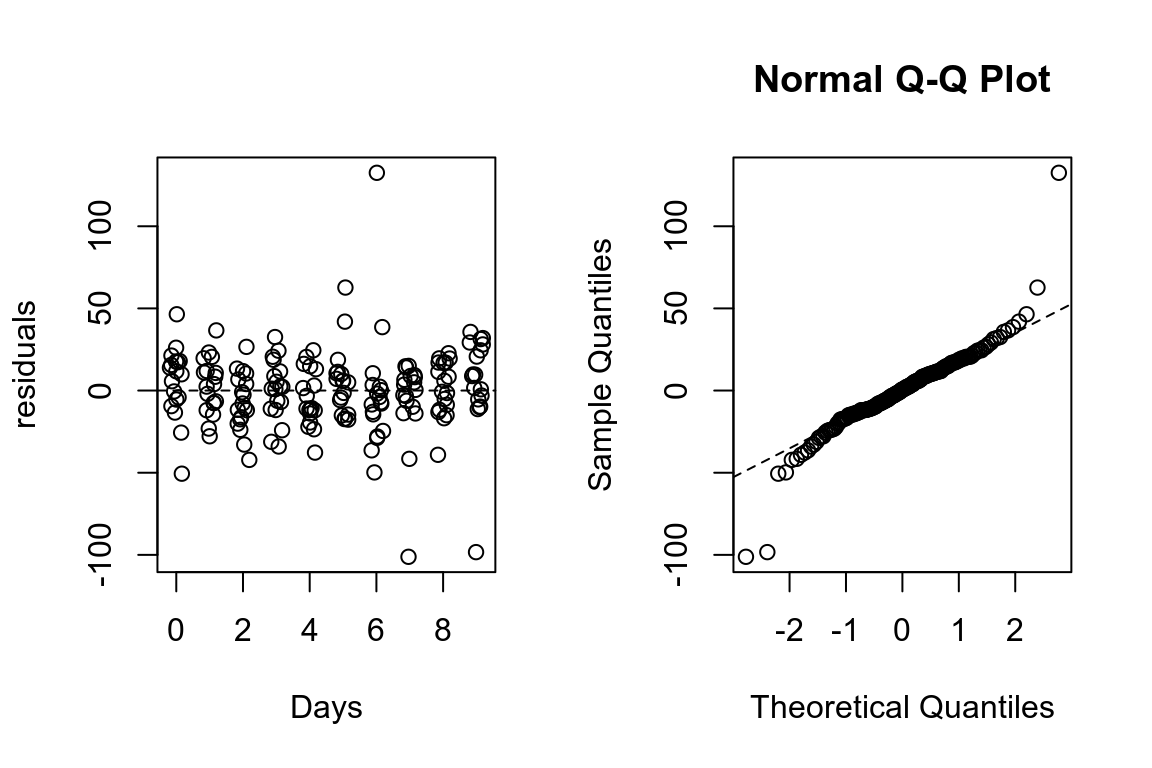## The frequentist approach • So far, we've seen multilevel models from a frequentist point of view. • parameters are seen as unknown fixed quantities. • the goal is to find best guesses (point-estimates) of the parameters. • uncertainty in the parameter's value is not treated probabilistically. • uncertainty in the estimates is characterized in relation to the data-generating process (think about the highly un-intuitive definition of confidence interval). • probability is interpreted as the expected long-run frequency of an event in an (imaginary) very large sample. ## The Bayesian approach • What is different in the Bayesian approach? • probability is interpreted as the degree of belief in the occurrence of an event, or in a proposition. • parameters are seen as unknown random variable, uncertainty in their values represented by a prior probability distribution. • the goal is to update the prior distribution on the basis of the available data, leading to a posterior probability distribution. • this is done with Bayes' theorem, however the Bayesian approach is not distinguished by Bayes' theorem (which is just a trivial implication of probability theory). • "The essential characteristic of Bayesian methods is their explicit use of probability for quantifying uncertainty in inferences based on statistical analysis" (Gelman et al. 2013). ## Bayesian models • In a typical Bayesian setting we have a likelihood $$p\left(\text{data} \mid \theta\right)$$, conditional on the parameter $$\theta$$, and a prior distribution $$p\left(\theta\right)$$. The posterior distribution for the parameter is $p\left(\theta \mid \text{data}\right) = \frac{p\left(\text{data} \mid \theta\right) p\left(\theta\right)}{p\left( \text{data} \right)}$ ## Bayesian models • In a typical Bayesian setting we have a likelihood $$p\left(\text{data} \mid \theta\right)$$, conditional on the parameter $$\theta$$, and a prior distribution $$p\left(\theta\right)$$. The posterior distribution for the parameter is $p\left(\theta \mid \text{data}\right) = \frac{p\left(\text{data} \mid \theta\right)p\left(\theta\right)}{\int p\left( \text{data} \mid \theta \right) p\left(\theta\right) d\theta}$ ## Bayesian models • In a typical Bayesian setting we have a likelihood $$p\left(\text{data} \mid \theta\right)$$, conditional on the parameter $$\theta$$, and a prior distribution $$p\left(\theta\right)$$. The posterior distribution for the parameter is $p\left(\theta \mid \text{data} \right) = \frac{p\left(\text{data} \mid \theta\right)p\left(\theta\right)}{\int p\left( \text{data} \mid \theta \right) p\left(\theta\right) d\theta}\\ \text{posterior} = \frac{\text{likelihood} \times \text{prior}}{\text{average likelihood}}$ ## Bayesian models • In a typical Bayesian setting we have a likelihood $$p\left(\text{data} \mid \theta\right)$$, conditional on the parameter $$\theta$$, and a prior distribution $$p\left(\theta\right)$$. The posterior distribution for the parameter is $p\left(\theta \mid \text{data}\right) \propto p\left(\text{data} \mid \theta\right)p\left(\theta\right)\\ \text{posterior} \propto \text{likelihood} \times \text{prior}$ ## Bayesian multilevel models • In the case of a multilevel model, each cluster $$j$$ has parameter $$\theta_j$$ which is an independent sample from a population distribution governed by some hyperparameter $$\phi$$. • $$\phi$$ is also not known, and thus has its prior distribution $$p\left(\phi\right)$$ (hyperprior). • We have a joint prior distribution: $$p\left(\phi,\theta\right)=p\left(\phi\right) \prod_{j=1}^{J} p\left(\theta_j \mid \phi\right)$$ • and a joint posterior distribution: $p\left(\phi,\theta \mid \text{data}\right) \propto \underbrace{p\left(\phi\right) \prod_{j=1}^{J} p\left(\theta_j \mid \phi\right)}_\text{prior} \times \underbrace{ p\left(\text{data} \mid \theta_j\right)}_\text{likelihood}$ ## Bayesian parameter inference • The information we want about a parameter $$\phi$$ is contained in its marginal posterior distribution $$p\left(\phi \mid \text{data} \right)=\int p\left(\phi,\theta \mid \text{data} \right) d\theta$$. • The calculation can be difficult once a model has many parameters (to describe the uncertainty in a parameter of interest one must average or marginalise over the uncertainty in all other parameters). • Monte Carlo techniques and computer simulations (Markov-Chain Monte Carlo sampling, MCMC) can be used to draw samples from the posterior distribution. • Once you have samples from the posterior distribution, summarizing the marginal posterior distribution becomes simply a matter of counting values. ## Metropolis algorithm Say you want to sample from a density $$P({\bf x})$$, but you can only evaluate a function $$f({\bf x})$$ that is proportional to the density $$P({\bf x})$$: • Initialization: 1. choose arbitrary starting point $${\bf x}_0$$ 2. choose a probability density to generate proposals $$g({\bf x}_{n+1}|{\bf x}_n)$$ (proposal density) • For each iteration $$i$$: 1. generate a candidate $${\bf x'}$$ sampling from $$g({\bf x}_{i+1}|{\bf x}_{i})$$ 2. calculate the acceptance ratio $$a = \frac{f({\bf x}')}{f({\bf x}_{i})}$$ 3. accept/reject: generate a uniform number $$u$$ in $$[0,1]$$ • if $$u \le a$$, accept and set $${\bf x}_{i+1}={\bf x'}$$ ("move to the new value") • if $$u > a$$, reject and set $${\bf x}_{i+1}={\bf x}_{i}$$ ("stay where you are") ## MCMC example Let use the sampling algorithm to infer values from one participant of the dataset sleepstudy.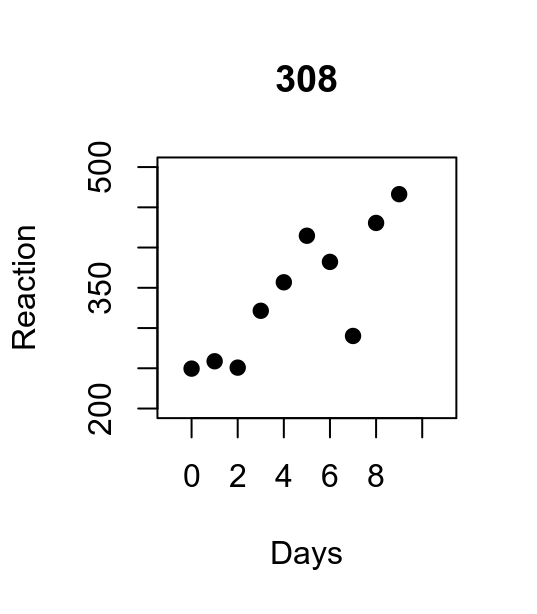• To sample from the posterior distribution of parameters is sufficient to compute the un-normalized posterior: $$\text{prior} \times \text{likelihood}$$ ## MCMC example First, code one function to compute the (log)likelihood, keeping the data fixed. x <- sleepstudy$Days[sleepstudy$Subject == "308"] y <- sleepstudy$Reaction[sleepstudy\$Subject == "308"]

loglik <- function(par) {
# par = [intercept, slope, log(residual Std.)]
pred <- par + par * x
return(sum(dnorm(y, mean = pred, sd = exp(par), log = T)))
}

## MCMC example